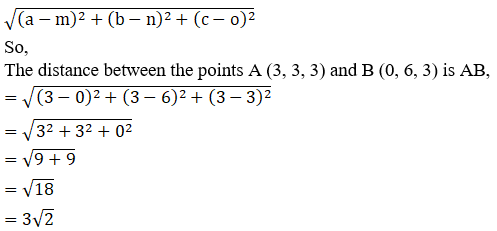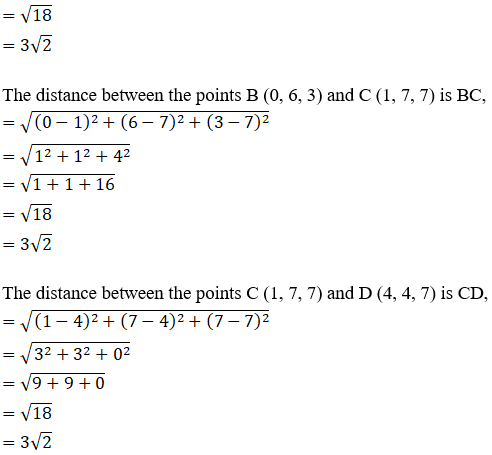• +91 9971497814
• info@interviewmaterial.com

# RD Chapter 28- Introduction to 3D coordinate geometry Ex-28.2 Interview Questions Answers

### Related Subjects

Question 1 :
Find the distance between the following pairs of points:
(i) P(1, -1, 0) and Q (2, 1, 2)
(ii) A(3, 2, -1) and B (-1, -1, -1)

(i) P(1,-1, 0) and Q (2, 1, 2)

Given:

The points P(1, -1, 0) and Q (2, 1, 2)

By using the formula,

The distance between any two points (a, b, c) and (m, n, o) isgiven by,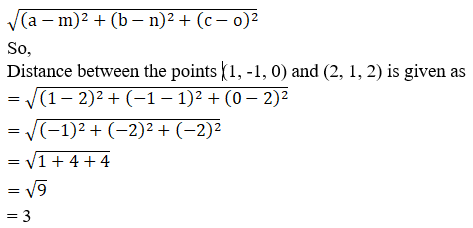TheDistance between P and Q is 3 units.

(ii) A (3,2, -1) and B (-1, -1, -1)

Given:

The points A (3, 2, -1) and B (-1, -1, -1)

By using the formula,

The distance between any two points (a, b, c) and (m, n, o) isgiven by,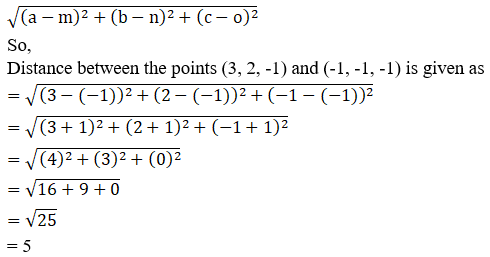= 5

TheDistance between A and B is 5 units.

Question 2 : Find the distance between the points P and Q having coordinates (-2, 3, 1) and (2, 1, 2).

Given:

The points (-2, 3, 1) and (2, 1, 2)

By using the formula,

The distance between any two points (a, b, c) and (m, n, o) isgiven by,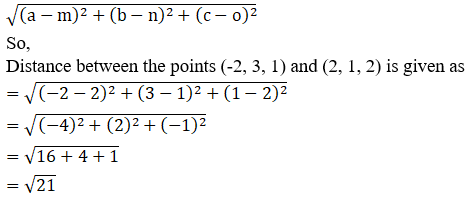TheDistance between the given two points is 21units.

Question 3 :
Using distance formula prove that the following points are collinear:
(i) A(4, -3, -1), B(5, -7, 6) and C(3, 1, -8)
(ii) P(0, 7, -7), Q(1, 4, -5) and R(-1, 10, -9)
(iii) A(3, -5, 1), B(-1, 0, 8) and C(7, -10, -6)

(i) A(4,-3, -1), B(5, -7, 6) and C(3, 1, -8)

Given:

The points A(4, -3, -1), B(5, -7, 6) and C(3, 1, -8)

Points A, B and C are collinear if AB + BC = AC or AB + AC = BCor AC + BC = AB

By using the formula,

The distance between any two points (a, b, c) and (m, n, o)is given by,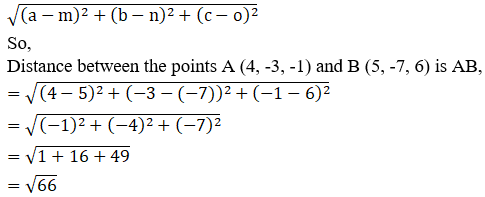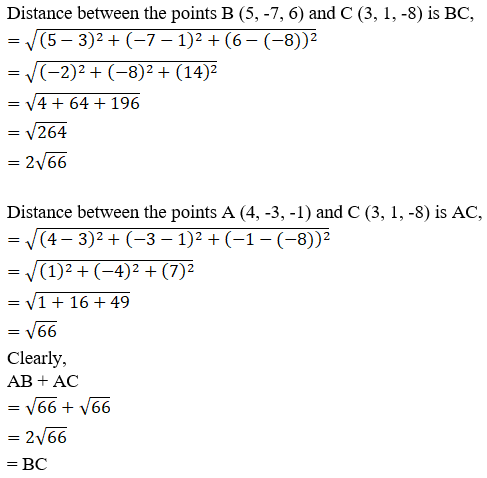Thepoints A, B and C are collinear.

(ii) P (0,7, -7), Q (1, 4, -5) and R (-1, 10, -9)

Given:

The points P (0, 7, -7), Q (1, 4, -5) and R (-1, 10, -9)

Points P, Q and R are collinear if PQ + QR = PR or PQ + PR = QRor PR + QR = PQ

By using the formula,

Distance between any two points (a, b, c) and (m, n, o) is givenby,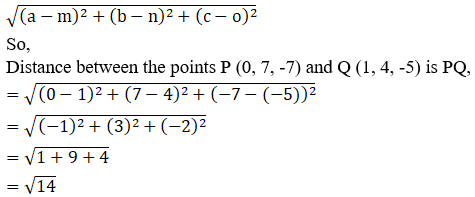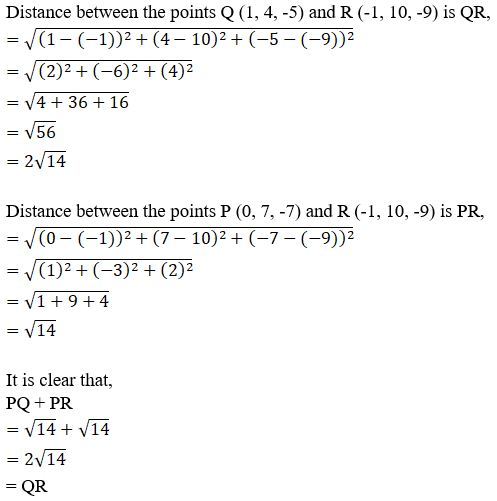Thepoints P, Q and R are collinear.

(iii) A(3,-5, 1), B(-1, 0, 8) and C(7, -10, -6)

Given:

The points A(3, -5, 1), B(-1, 0, 8) and C(7, -10, -6)

Points A, B and C are collinear if AB + BC = AC or AB + AC = BCor AC + BC = AB

By using the formula,

The distance between any two points (a, b, c) and (m, n, o)is given by,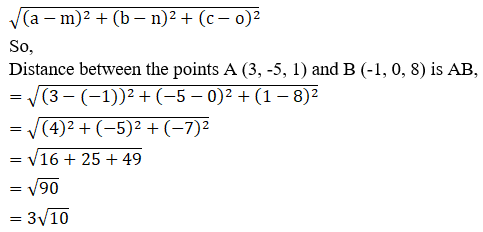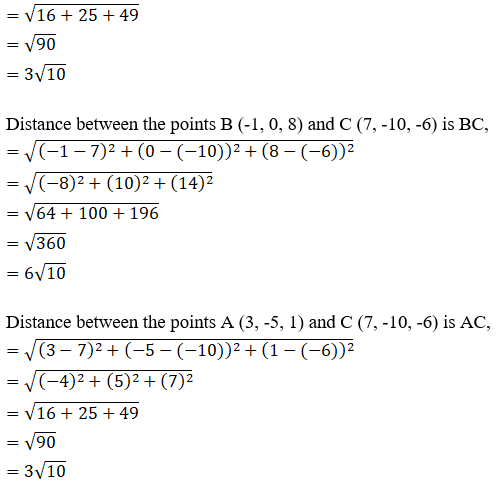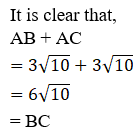Thepoints A, B and C are collinear.

Question 4 : Determine the points in (i) xy-plane (ii) yz-plane and (iii) zx-plane which are equidistant from the points A(1, -1, 0), B(2, 1, 2) and C(3, 2, -1).

Given:
The points A(1, -1, 0), B(2, 1, 2) and C(3, 2, -1)
(i) xy-plane
We know z = 0 in xy-plane.
So let P(x, y, 0) be any point in xy-plane
According to the question:
PA = PB = PC
PA2 = PB2 = PC2
By using the formula,
The distance between any two points (a, b, c) and (m, n, o) is given by,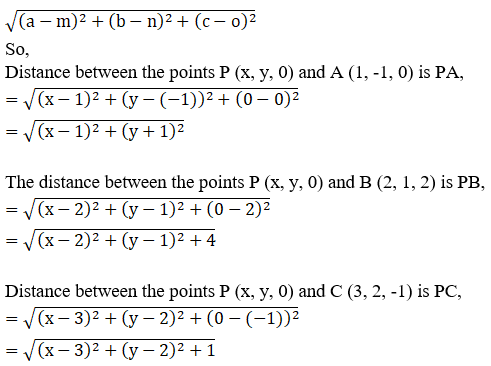We know PA2 = PB2

So, (x – 1)2+ (y + 1)2 = (x – 2)2 +(y – 1)2 + 4

x2+ 1 – 2x + y2 + 1 + 2y = x2+4 – 4x + y2 + 1 – 2y + 4

– 2x + 2 + 2y = 9 – 4x – 2y

– 2x + 2 + 2y – 9 + 4x + 2y = 0

2x + 4y – 7 = 0

2x = – 4y + 7……………………(1)

Since, PA2 = PC2

So, (x – 1)2+ (y + 1)2 = (x – 3)2 +(y – 2)2 + 1

x2+ 1 – 2x + y2 + 1 + 2y = x2+9 – 6x + y2 + 4 – 4y + 1

– 2x + 2 + 2y = 14 – 6x – 4y

– 2x + 2 + 2y – 14 + 6x + 4y = 0

4x + 6y – 12 = 0

2(2x + 3y – 6) = 0

Now substitute the value of 2x (obtained in equation (1)), weget

7 – 4y + 3y – 6 = 0

– y + 1 = 0

y = 1

By substituting the value of y back in equation (1) we get,

2x = 7 – 4y

2x = 7 – 4(1)

2x = 3

x = 3/2

Thepoint P (3/2, 1, 0) in xy-plane is equidistant from A, B and C.

(ii) yz-plane

We know x = 0 in yz-plane.

Let Q(0, y, z) any point in yz-plane

According to the question:

QA = QB = QC

QA2 = QB2 = QC2

By using the formula,

The distance between any two points (a, b, c) and (m, n, o)is given by,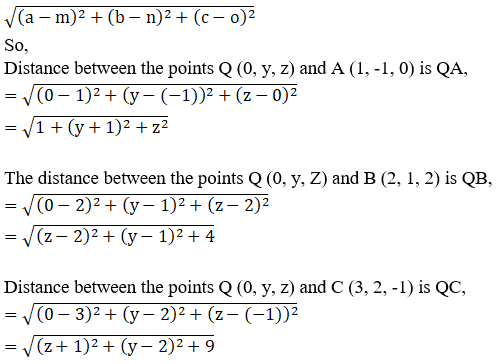We know, QA2 = QB2

So, 1 + z2+ (y + 1)2 = (z – 2)2 +(y – 1)2 + 4

z2+ 1 + y2 + 1 + 2y = z2+4 – 4z + y2 + 1 – 2y + 4

2 + 2y = 9 – 4z – 2y

2 + 2y – 9 + 4z + 2y = 0

4y + 4z – 7 = 0

4z = –4y + 7

z = [–4y + 7]/4 …. (1)

Since, QA2 = QC2

So, 1 + z2+ (y + 1)2 = (z + 1)2 +(y – 2)2 + 9

2+ 1 + y2 + 1 + 2y = z2+ 1 + 2z + y2 +4 – 4y + 9

2 + 2y = 14 + 2z – 4y

2 + 2y – 14 – 2z + 4y = 0

–2z + 6y – 12 = 0

2(–z + 3y – 6) = 0

Now, substitute the value of z [obtained from (1)] we get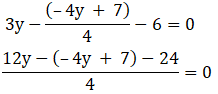12y + 4y – 7 – 24 = 0

16y – 31 = 0

y = 31/16

Substitute the value of y back in equation (1), we get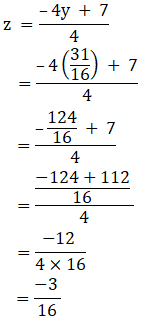Thepoint Q (0, 31/16, -3/16) in yz-plane is equidistant from A, B and C.

(iii) zx-plane

We know y = 0 in xz-plane.

Let R(x, 0, z) any point in xz-plane

According to the question:

RA = RB = RC

RA2 = RB2 = RC2

By using the formula,

The distance between any two points (a, b, c) and (m, n, o)is given by,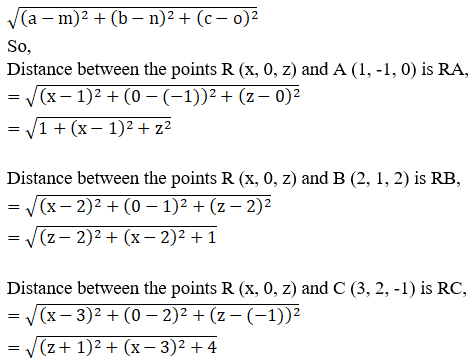We know, RA2 = RB2

So, 1 + z2+ (x – 1)2 = (z – 2)2 +(x – 2)2 + 1

z2+ 1 + x2 + 1 – 2x = z2+4 – 4z + x2 + 4 – 4x + 1

2 – 2x = 9 – 4z – 4x

2 + 4z – 9 + 4x – 2x = 0

2x + 4z – 7 = 0

2x = –4z + 7……………………………(1)

Since, RA2 = RC2

So, 1 + z2+ (x – 1)2 = (z + 1)2 +(x – 3)2 + 4

z2+ 1 + x2 + 1 – 2x = z2+1 + 2z + x2 + 9 – 6x + 4

2 – 2x = 14 + 2z – 6x

2 – 2x – 14 – 2z + 6x = 0

–2z + 4x – 12 = 0

2(2x) = 12 + 2z

Substitute the value of 2x [obtained from equation (1)] we get,

2(–4z + 7) = 12 + 2z

–8z + 14 = 12 + 2z

14 – 12 = 8z + 2z

10z = 2

z = 2/10

= 1/5

Now, substitute the value of z back in equation (1), we get

2x = -4z + 7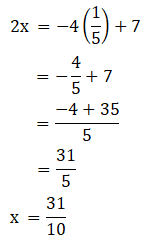Thepoint R (31/10, 0, 1/5) in xz-plane is equidistant from A, B and C.

Question 5 : Determine the point on z-axis which is equidistant from the points (1, 5, 7) and (5, 1, -4)

Given:
The points (1, 5, 7) and (5, 1, -4)
We know x = 0 and y = 0 on z-axis
Let R(0, 0, z) any point on z-axis
According to the question:
RA = RB
RA2 = RB2
By using the formula,
The distance between any two points (a, b, c) and (m, n, o) is given by,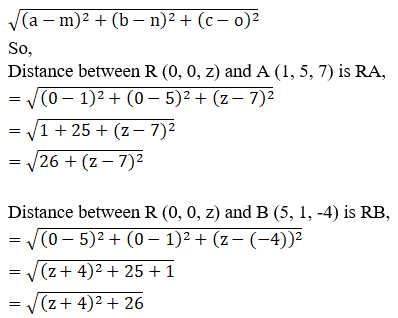We know, RA2 = RB2

26+ (z – 7)2 = (z + 4)2 + 26

z2+ 49 – 14z + 26 = z2+ 16 + 8z + 26

49 – 14z = 16 + 8z

49 – 16 = 14z + 8z

22z = 33

z = 33/22

= 3/2

Thepoint R (0, 0, 3/2) on z-axis is equidistant from (1, 5, 7) and (5, 1,-4).

Question 6 : Find the point on y-axis which is equidistant from the points (3, 1, 2) and (5, 5, 2).

Given:
The points (3, 1, 2) and (5, 5, 2)
We know x = 0 and z = 0 on y-axis
Let R(0, y, 0) any point on the y-axis
According to the question:
RA = RB
RA2 = RB2
By using the formula,
The distance between any two points (a, b, c) and (m, n, o) is given by,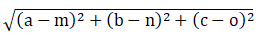So,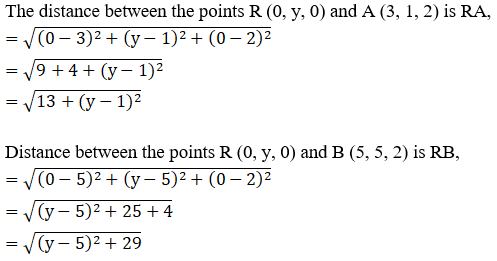We know, RA2 = RB2

13+ (y – 1)2 = (y – 5)2 + 29

y2+ 1 – 2y + 13 = y2+ 25 – 10y + 29

10y – 2y = 54 – 14

8y = 40

y = 40/8

= 5

Thepoint R (0, 5, 0) on y-axis is equidistant from (3, 1, 2) and (5, 5, 2).

Question 7 : Find the points on z-axis which are at a distance√21 from the point (1, 2, 3).

Given:

The point (1, 2, 3)

Distance = √21

We know x = 0 and y = 0 on z-axis

Let R(0, 0, z) any point on z-axis

According to question:

RA = √21

RA2 = 21

By using the formula,

The distance between any two points (a, b, c) and (m, n, o)is given by,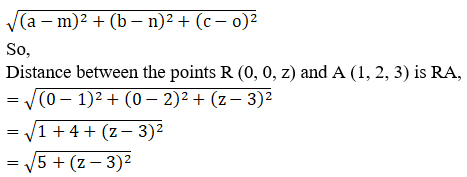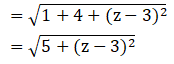We know, RA2 = 21

5 + (z – 3)2 = 21

z2+ 9 – 6z + 5 = 21

z2 – 6z = 21 – 14

z2– 6z – 7 = 0

z2– 7z + z – 7 = 0

z(z– 7) + 1(z – 7) = 0

(z– 7) (z + 1) = 0

(z– 7) = 0 or (z + 1) = 0

z= 7 or z = -1

The points(0, 0, 7) and (0, 0, -1) on z-axis is equidistant from (1, 2, 3).

Question 8 : Prove that the triangle formed by joining the three points whose coordinates are (1, 2, 3), (2, 3, 1) and (3, 1, 2) is an equilateral triangle.

Given:
The points (1, 2, 3), (2, 3, 1) and (3, 1, 2)
An equilateral triangle is a triangle whose all sides are equal.
So let us prove AB = BC = AC
By using the formula,
The distance between any two points (a, b, c) and (m, n, o) is given by,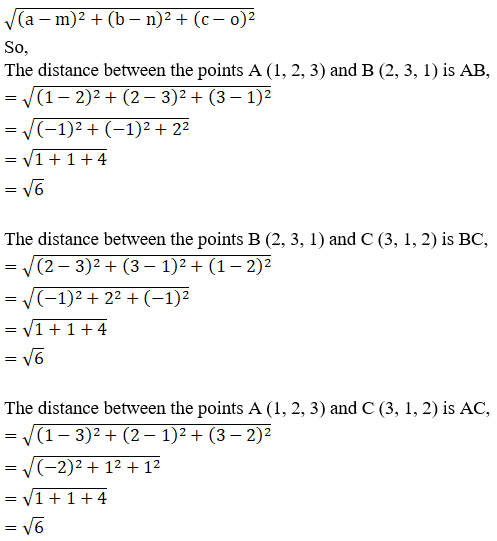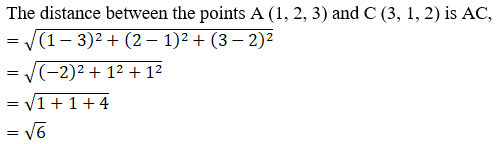It is clear that,

AB = BC = AC

Δ ABC is a equilateral triangle

Hence Proved.

Question 9 : Show that the points (0, 7, 10), (-1, 6, 6) and (-4, 9, 6) are the vertices of an isosceles right-angled triangle.

Given:

The points (0, 7, 10), (-1, 6, 6) and (-4, 9, 6)

Isosceles right-angled triangle is a triangle whose two sidesare equal and also satisfies Pythagoras Theorem.

By using the formula,

The distance between any two points (a, b, c) and (m, n, o)is given by,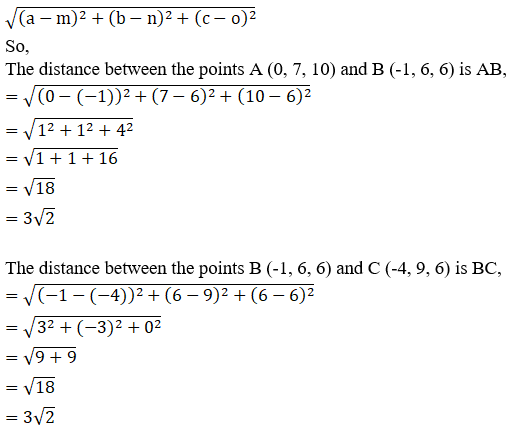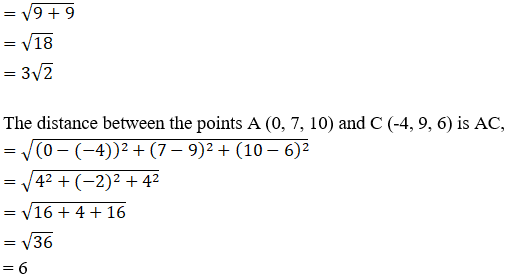Since, AB = BC

So, AB2 + BC2

= (32)2 +(32)2

= 18 + 18

= 36

= AC2

We know that, AB = BC and AB2 + BC2 = AC2

So, Δ ABC is an isosceles-right angled triangle

Hence Proved.

Question 10 : Show that the points A(3, 3, 3), B(0, 6, 3), C(1, 7, 7) and D(4, 4, 7) are the vertices of squares.